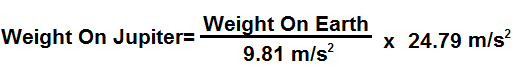﻿ Weight On Jupiter Calculator ﻿# Weight on Jupiter Calculator### How Much Would You Or Any Object Weigh On Jupiter?Enter Weight: Any unit grams (g) kilograms (kg) pounds (lbs)

This Weight on Jupiter Conversion Calculator calculates your weight or the weight that any object would be if on Jupiter.

To use this calculator, a user just enters his or her weight or the object's weight and clicks the 'Calculate' button, and the weight will automatically be computed and shown below. The weight can be entered in any units and the resultant answer will be in the same units which the user has input. Thus, for example, if a user enters 210lbs, the resultant answer of the weight in Jupiter would be 530.67lbs. The units will always match. Just for the sake of clarity, a user can select the units he wants the answer to appear in, whether grams, kilograms, or pounds, and that unit will show in the answer.

This calculator calculates the weight on Jupiter from the weight of the object on Earth. Being that it takes the weight of an object on Earth and converts it to the weight on Jupiter, the formula is Weight on Jupiter= (Weight on Earth/9.81m/s2) * 24.79m/s2. To find the weight on Jupiter, we divide the weight on Earth by the Earth's force of gravity, which is 9.81m/s2. This calculates the mass of the object. Once we have the object's mass, we can find the weight by multiplying it by the gravitational force, which it is subject to. Being that Jupiter has a gravitational force of 24.79m/s2, we multiply the object's mass by this quanitity to calculate an object's weight on Jupiter.

So an object or person on Jupiter would weigh 252.70% of its weight on Earth. Therefore, a person would be heavier on Jupiter than on Earth. Conversely, a person is 39.6% lighter on Earth than on Jupiter.

What causes the differences in weight between the various planets? The answer is the gravitational force which a planet is subject to. Earth has less mass than Jupiter. Therefore, the sun pulls on the Earth with lesser force, because of the lesser mass. You probably are familiar that gravity is a downward force. It pushes you back to the Earth's surface. This is why when you jump, you come back down. Being that the Earth has lesser gravitational force, it pulls object downward with lesser force. This translates into lesser weight.

If you want to calculate the weight of all of the planets simultaneously, see our Weight on Planets Calculator.

Related Resources

﻿